# Numerical Study of Natural Convection Heat Transfer in Porous Media Square Cavity with Multiple Cold Walls Based on LBM

Numerical Study of Natural Convection Heat Transfer in Porous Media Square Cavity with Multiple Cold Walls Based on LBM

Zheshu MaLuobin Duan Shouguang Yao Xinwang Jia

College of Automobile and Traffic Engineering, Nanjing Forestry University, Nanjing, Jiangsu, 210037, China

School of Energy and Power Engineering, Jiangsu University of Science and Technology, Zhenjiang, Jiangsu 212003, China

Corresponding Author Email:
mazheshu@126.com
Page:
69-76
|
DOI:
http://dx.doi.org/10.18280/ijht.330409
|
Accepted:
|
Published:
31 December 2015
| Citation

OPEN ACCESS

Abstract:

In the present work, the natural convection problem in a two-dimensional square cavity filled with porous media is simulated by Lattice Boltzmann method(LBM). The research cavity is characterized by three cooling sides, two cold vertical walls whose size is varied, and the last side heated from below.The flow field and temperature field of fluid in the porous cavity under condition of different cooling size of both left and right wall are obtained by numerically simulating. A comprehensive study of the effect of natural convection heat transfer is carried out for various values of the length of the dimensionless D , of Rayleigh number, of Darcy number, and of porosity. The results show that we should make it more than half the length of the vertical surface when setting the cold vertical walls size. Thus, the intensity of natural convection heat transfer will enhance.Conversely, the convection effect is not obvious.High Rayleigh number and Darcy number has stronger effect on natural convection and in the case of high Darcy number, the changing of porosity has obvious influence on heat transfer.

(Presented at the AIGE Conference 2015)

Keywords:

Natural convection, LBM, Porous media, Numerical simulation.

1. Introduction

Porous media is a heterogeneous object which is composed of solid skeleton and tiny gaps filled with single phase or multiphase coexistence fluids between skeletons.The phenomena of energy, momentum and mass transfer in porous media exist in every field of industrial and agricultural production. Natural convection heat transfer in porous media is a benchmark problem due to its wide application background, such as heat pipe, heat preservation material, oil storage industry, decontamination of groundwater, thermal drying and casting solidification, etc. Ingham and Pop [1, 2] elaborated the natural convection problem in porous media in their works. An excellent and comprehensive review has been given by Nield and Bejan . The numerical method for solving the convection problem of porous media and the knowledge of engineering applications are extensively investigated by other scholars [4～6], and they broadened the study in this field.

As a promising numerical method, Lattice Boltzmann method(LBM) [7～9] has became a new tool to simulate fluid motion and model complex physical phenomena after decades of development. Different from the traditional method of computational fluid mechanics, LBM is not based on the macroscopic continuous equation but grounded on the fluid microscopic model and mesoscopic dynamic equations. Then, the evolution mechanism in accord with physical laws is constructed to calculate. LBM can be used to simulate the fluid flows and heat transfer due to its simple implements, good concurrency, simple boundary treatment etc, and it also has been widely employed to study of the works in porous media by international scholars. Seta et al.  applied lattice Boltzmann method to analyze the performance of natural convection heat transfer in porous media cavity for different Rayleigh number, Darcy number and porosity. Yan  et al. [11, 12] implemented LBM to simulate the flow field and the temperature field in a cavity filled with porous medium, especially researched the influence of the porosity and the porosity of the medium vary from place to place on the nature convection.Xun  studied the natural convection problem in a two-dimensional cavity that the upper horizontal wall and the vertical walls were held at a constant cold temperature, and another wall was heated locally from below. It investigated the change of flow field and temperature field by adjusting heat wall length and Rayleigh number.

In actual engineering, the natural convection heat transfer problem in a porous media cavity which is heated from below and the size of two cold vertical walls is varied has wide applications, such as equipment cooling, heat preservation, drying processes, etc. Therefore, the study of the problem has an important significance. This paper uses the coupled lattice Boltzmann model proposed by Guo et al.  To solve the heat transfer problem in porous media at representative elementary volume scale by writing C++ program. On this basis, a comprehensive study of the effect of natural convection heat transfer is carried out for various values of the length of the dimensionless $D$, of Rayleigh number, of Darcy number, and of porosity.

2. Physical Model and Governing Equations

2.1 Physical model

In this paper, the physical model is a two-dimensional square cavity of length $L$ that filled with porous media, as shown in Figure 1. In the model, the vertical surfaces are partially held at a constant temperature $T_{\mathrm{L}}$ and remaining impermeable walls are adiabatic. The actual length of the cold walls is $d$ and the dimensionless length of the walls is $D(d / L)$ . The horizontal walls are under constant temperature $T_{\mathrm{H}}$, at $y=0$ and $T_{\mathrm{L}}$ , at $y=L$ , respectively. The origin of coordinate system is the lower left corner of the cavity, and take horizontal direction as the $x$ direction, the opposite direction of gravity as the $y$ direction.

## 1.png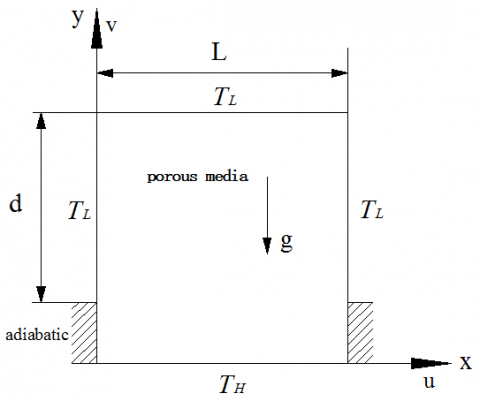Figure 1. Schematic of the physical model of enclosure cavity

The initial conditions and boundary conditions are as follows:

$t=0, \quad u=v=0, \quad T=0$ ;

$x=0, \quad u=v=0, \quad T=T_{L}$ ;

$x=0, \quad u=v=0, \quad \frac{\partial T}{\partial x}=0$ ; (adiabatic section)

$x=L, \quad u=v=0, \quad T=T_{L}$ ;

$x=L, \quad u=v=0, \quad \frac{\partial T}{\partial x}=0$ ; (adiabatic section)

$y=0, \quad u=v=0, \quad T=T_{H}$ ;

$y=L, \quad u=v=0, \quad T=T_{L}$ .

2.2 Governing equations

In the study, we assume that the configuration of porous media in the cavity is homogeneous, rigid and isotropic. We also assume that the convective incompressible, and viscous fluid flow is described by Brinkman-Forchheimer model and that the Boussinesq approximation is valid. At this point, the flow continuity equation and Brinkman-Forchheimer equation can be written as the following form :

$\nabla \cdot \boldsymbol{u}=0$  (1)

$\frac{\partial \boldsymbol{u}}{\partial t}+(\boldsymbol{u} \cdot \nabla)\left(\frac{\boldsymbol{u}}{\varepsilon}\right)=-\frac{1}{\rho} \nabla(\varepsilon p)+v_{e} \nabla^{2} \boldsymbol{u}+\boldsymbol{F}$      (2)

Where, $\varepsilon$ is the porosity of porous media, $\rho$ is the density of fluid, $u$ and $p$ is the average speed of fluid volume and pressure, respectively, $v_{e}$ is the effective kinematic viscosity coefficient. $\boldsymbol{F}$ is the total body force due to the presence of a porous media and other external force fields, it can be written as the following form

$\boldsymbol{F}=-\frac{\varepsilon v}{K} \boldsymbol{u}-\frac{\varepsilon F_{\varepsilon}}{\sqrt{K}}|\boldsymbol{u}| \boldsymbol{u}+\varepsilon \boldsymbol{G}$  (3)

On the right-hand side of the equation, the first item is the frictional resistance of fluid and porous media skeleton, and the second one is the inertia due to the presence of a porous medium. $v$ is the kinematic viscosity of the fluid, $K$ is the permeability and F_{\varepsilon} represents the geometric function, $G$ is the volume force caused by external forces.If $G$ is caused only by gravity, under the Boussinesq assumption, the influence of gravity can be expressed as

$\boldsymbol{G}=-\boldsymbol{g} \beta\left(T-T_{m}\right)$    (4)

Where, $\boldsymbol{g}$ is the gravitational acceleration, $\beta$ is the thermal expansion coefficient, and $T_{m}$ is the average temperature of the system.The geometric function $F_{\varepsilon}$ and the permeability $K$ have relationship with the porosity $\mathcal{E}$, respectively.For the porous media that is made of solid particles, $F_{\varepsilon}$ and $K$ can be expressed based on Ergun’s  empirical formula as

$F_{\varepsilon}=\frac{1.75}{\sqrt{150 \varepsilon^{3}}}$ , $K=\frac{\varepsilon^{3} d_{p}^{2}}{150(1-\varepsilon)^{2}}$     (5)

Where $d_{p}$ is the diameter of the solid particles.

When heat transfer problem is involved in fluid flow, if we ignore the compression work and the viscous heat dissipation, it can meet local thermodynamic equilibrium condition between the fluid and  solid, then the energy equation of convection heat transfer in the porous media can be expressed as

$\sigma \frac{\partial T}{\partial t}+\boldsymbol{u} \cdot \nabla T=\nabla \cdot\left(\alpha_{m} \nabla T\right)$     (6)

Where $T$ is the average volume temperature of fluid, $\sigma=\varepsilon+(1-\varepsilon) \rho_{s} c_{p s} / \rho_{f} c_{p f}$ represents the ratio between the heat capacities of the solid and fluid phase, $\rho_{s}$ , $\rho_{f}$ , $C_{p s}$ , $C_{p f}$  are the density and capacity of the solid and fluid phase, respectively, $\alpha_{m}$ denotes the effective thermal diffusivity.

In order to represent the characters of natural convection heat transfer in porous media, we can introduce several dimensionless numbers and its exprssion: the Darcy number $D a=K / L^{2}$ , the viscosity ratio $J=v_{e} / v$ , the Prandtl number $\operatorname{Pr}=\boldsymbol{v} / \alpha_{m}$ , and the Rayleigh number $R a=g \beta \Delta T L^{3} / v \alpha_{m}$ .

Where $L$ is the cavity length, $\Delta T=T_{H}-T_{L}$ is the temperature difference between the hot and cold side walls.

3. Lattice Boltzmann Model

For the natural convection heat transfer problems in porous media in this paper, we use the double distribution function model to study the flow field and temperature field of fluid.Meanwhile, the D2Q9 model is accepted and the Lattice Boltzmann evolution equations can be expressed as follows:

$f_{i}\left(\boldsymbol{x}+\boldsymbol{e}_{i} \delta t, t+\delta_{t}\right)-f_{i}(\boldsymbol{x}, t)=-\frac{f_{i}(\boldsymbol{x}, t)-f_{i}^{e q}(\boldsymbol{x}, t)}{\tau_{v}}+\delta_{t} F_{i}$    (7)

$g_{i}\left(\boldsymbol{x}+\boldsymbol{e}_{i} \delta t, t+\delta_{t}\right)-g_{i}(\boldsymbol{x}, t)=-\frac{g_{i}(\boldsymbol{x}, t)-g_{i}^{e q}(\boldsymbol{x}, t)}{\tau_{T}}$     (8)

Where, $i=0: 8$ ; $f_{i}$ is the distribution function; $f_{i}^{\mathrm{eq}}$ is the corresponding equilibrium distribution function; $g_{i}$ is the temperature distribution function and $g_{i}^{e q}$ is the equilibrium temperature distribution function; $\tau_{v}$ and $\tau_{T}$ is the velocity nondimensional relaxation time and the temperature relaxation time, respectively. Equation (7) recovers the continuity and the momentum Equations. (1) and (2). Equation (8) describes the evolution of the internal energy and leads to Equation (6).

Usually the speed configuration of D2Q9 model is accepted as follows:

$\boldsymbol{e}_{i}=\left\{\begin{array}{cc}(0,0) & i=0 \\ c\left(\cos \left[(i-1) \frac{\pi}{2}\right], \sin \left[(i-1) \frac{\pi}{2}\right]\right) & i=1,2,3,4 \\ \sqrt{2}\left(\cos \left[(2 i-1) \frac{\pi}{4}\right], \sin \left[(2 i-1) \frac{\pi}{4}\right]\right) & i=5,6,7,8\end{array}\right.$   (9)

Where, lattice speed $c=\delta_{x} / \delta_{t}$ , $\delta_{x}$ and $\delta_{y}$ are time step and the grid step. Generally the grid spaces on the directions of x and y are the same $\delta_{x}=\delta_{y}$ .

On the basis of the continuous Boltzmann equation, we can get the equilibrium distribution function according to discrete the time and space.It is defined as

$f_{i}^{e q}=\omega_{i} \rho\left[1+\frac{\boldsymbol{e}_{i} \cdot \boldsymbol{u}}{c_{s}^{2}}+\frac{\boldsymbol{u} \boldsymbol{u}:\left(\boldsymbol{e}_{i} \boldsymbol{e}_{i}-c_{s}^{2} \boldsymbol{I}\right)}{2 \varepsilon c_{s}^{4}}\right]$      (10)

$g_{i}^{e q}=\omega_{i} T\left[\sigma+\frac{e_{i} \cdot u}{c_{s}^{2}}\right]$      (11)

Where, $\omega_{0}=4 / 9$ $c_{s}=c / \sqrt{3}$ is the speed of sound; the values of the weight are given by , $\omega_{i}=1 / 9(i=1: 4)$ , $\omega_{i}=1 / 36(i=5: 8)$ .

In Equation. (7), the forcing term can be given by

$F_{i}=\omega_{i} \rho\left(1-\frac{1}{2 \tau_{v}}\right)\left[\frac{e_{i} \cdot F}{c_{s}^{2}}+\frac{u F:\left(e_{i} e_{i}-c_{s}^{2} I\right)}{\varepsilon c_{s}^{4}}\right]$       (12)

The corresponding effective viscosity and the effective thermal conductivity in macro equation are given by

$v_{e}=c_{s}^{2}\left(\tau_{v}-\frac{1}{2}\right) \delta_{t}$ , $\alpha_{m}=\sigma c_{s}^{2}\left(\tau_{t}-\frac{1}{2}\right) \delta_{t}$          (13)

The macroscopic quantities, fluid density and internal energy are defined as

$\rho=\sum_{i} f_{i}$ , $T=\sum_{i} g_{i} / \sigma$      (14)

The speed of the fluid is calculated by using a temporary speed, it can be written as

$\boldsymbol{u}=\frac{\boldsymbol{v}}{c_{0}+\sqrt{c_{0}^{2}+c_{1} | \boldsymbol{v}} |}$      (15)

Where, parameters $c_{0}$ and $c_{1}$ and $v$ are given by

$c_{0}=\frac{1}{2}\left(1+\varepsilon \frac{\delta_{t}}{2} \frac{v}{K}\right), \quad c_{1}=\varepsilon \frac{\delta_{t}}{2} \frac{F_{\varepsilon}}{\sqrt{K}}, \quad v=\sum_{i} f_{i} \frac{e_{i}}{\rho}+\frac{\delta_{t}}{2} \varepsilon G$   (16)

4. Results of Numerical Simulation and Analysis

4.1 Method validation

In order to test the reliability of the model and method, the lattice Boltzmann method was used to simulate natural convection in a two-dimensional square cavity filled with porous medium.

The vertical surfaces of the cavity are held at a constant high temperature and low temperature, respectively. The horizontal walls are adiabatic and impermeable. To evaluate the calculation results, the comparison of average Nusselt numbers on the hot wall between the existing literature data and the LBM results is tabulated in Table 1. Meanwhile, it shows good quantitative agreement.

Table 1. Comparison of average Nusselt numbers on the hot wall with other numerical results

 $R a$ $\varepsilon$ $D a$ $\operatorname{Pr}$ Literature data  LBM result  LBM result  Calculated value of this paper 103 0.4 10-4 1.0 1.010 —— 1.007 1.001 105 0.4 10-4 1.0 1.067 1.066 1.063 1.058 106 0.4 10-4 1.0 2.550 2.602 2.544 2.573

4.2 The influence of size change of two cold vertical walls on natural convection heat transfer

In order to study the effect of changes in the size of the vertical cold walls on natural convection heat transfer in the cavity, computational parameters are set as follows: $D a=10^{-3}, \operatorname{Pr}=0.7, J=1, \sigma=1, \varepsilon=0.4$ , and then calculate with D ranging from 0 to 1. Figure 2 is the fluid streamlines and isotherms diagram when steady-state is reached under different D. It can be clearly seen from Figure 2, flow field is two circulation cells along the center axis of symmetry when the value of D is greater than zero and less than  or equal to one.When both sides of the vertical cold

walls size decrease, the center of the circulation cells move upward. Its isotherms are arched distribution and the start point of the arch relates to the lowest point of the cold surface, at the moment, the strength of natural convection heat transfer is directly related to the size of the vertical cold walls. To compare and observe quantitatively, we can find from Figure 3 that the average Nusselt number on hot wall grows slowly when D is less than 0.5, while the slope of the curve shows that the Nusselt number grows more pronounced when D is greater than 0.5. In other words, when setting the sizes of the left and right side of cold walls, we must make sure that they should be greater than half the length of the vertical plane, as a result, natural convection heat transfer intensity can exponentially increase.

## 2a.png(a)

## 2b.png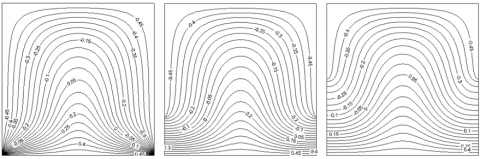(b)

D=1                                  D=0.75                                 D=0.5

Figure 2. Streamlines(a) and isotherms(b) for a variable $D \cdot D a=10^{-3} 、 \operatorname{Pr}=0.7 、 R a=10^{5}、\quad \varepsilon=0.4$ ; from left to right, D=1,0.75,0.5

## 3.png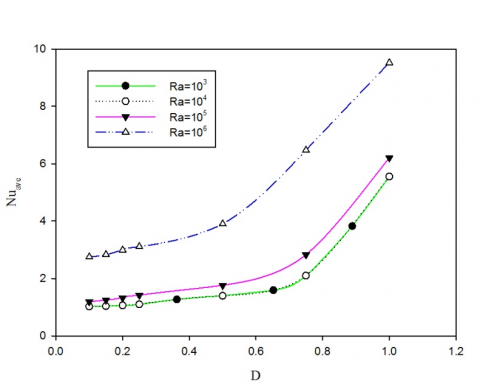Figure 3. Nusselt number as function of the dimensionless D for different Rayleigh numbers

4.3 The influence of Rayleigh number on natural convection heat transfer

Figure 4 shows the effects of different Rayleigh numbers on the average Nusselt number for $D a=10^{-3}, 10^{-6}, \varepsilon=0.4, \operatorname{Pr}=0.7, D=0.5、1.0$, the value of the Rayleigh numbers are 103, 104, 105 and 106, respectively. As can be seen from figure 4, with increasing $R a$, the average Nusselt numbers on the hot wall change obviously. When Ra<1e+5, the average Nusselt numbers on the hot wall increase gradually, when Ra>1e+5, the average Nusselt numbers jump significantly.This is because the larger the Rayleigh numbers are, the stronger the motivation of natural convection and vortex intensity will be. Conversely, at the low Rayleigh numbers, such as 103 to 104, the change of the hot wall average Nusselt number is not obvious. However, at a relative small Darcy number, the change of the Rayleigh number has little influence on the intensity of natural convection.For this time, the permeability of the cavity is small, the air circulation is poor, and the heat transfer is mainly depend on the solid heat conduction.

## 4.jpg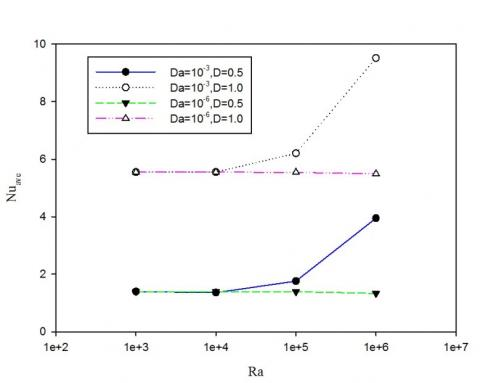Figure 4. The effects of Rayleigh number on the Nusselt number for $D a=10^{-3}, 10^{-6}, D=0.5,1.0$

4.4 The influence of Darcy number on natural convection heat transfer

Figure 5 shows the effects of different Darcy numbers on average Nusselt number. Under the condition of $R a=10^{5}, \quad \varepsilon=0.4, \quad \operatorname{Pr}=0.7, \quad D=0.5$ , the value of the Darcy numbers are 10-3, 10-4, 10-5, 10-6 and 10-7, respectively. With increasing $D a$, the average Nusselt number on hot wall increases greatly. However, when the Darcy number in the range of 10-4, the curve remains horizontal and the Nusselt number has a little growth. At $D a>10^{-4}$ , the Nusselt number increases rapidly. This is because of the existence of natural convection and heat conduction in the porous media cavity at the same time. At $D a<10^{-4}$ , with a poor permeability and air circulation, the heat transfer mainly rely on solid heat conduction. On the contrary, the higher values of Nusselt number for $D a>10^{-4}$ are a result of an increase in convection due to an increase in permeability.

## 5.jpg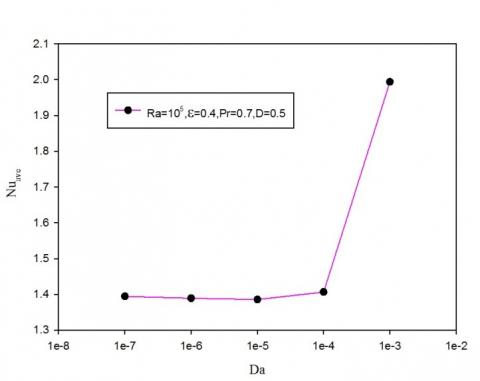Figure 5. The effects of Darcy number on the Nusselt number

4.5 The influence of porosity on natural convection heat transfer

Figure 6 shows the influence of the porosity on heat transfer with different Darcy numbers at $R a=10^{5}, \operatorname{Pr}=0.7, \quad D=0.5$ . Here we can see that,  the convection within the cavity at low $D a$, the variation of porosity has very little impact on the average Nusselt number on the hot wall. At $D a \geq 10^{-3}$ , the influence of the porosity on natural convection is significant .

## 6.jpg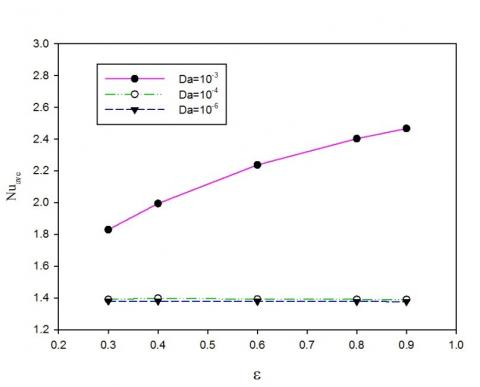Figure 6. Nusselt number as function of the porosity for different Darcy numbers

5. Conclusion

A systematic numerical study of natural convection in the cavity filled with porous media which is heated from below and the size of two cold vertical walls is varied has been presented. By using the lattice Boltzmann method, the influence of  dimensionless length $D$ , Rayleigh number, Darcy number, and porosity on natural convection is considered. The simulation results lead to the following conclusions:

(1)The lattice Boltzmann method and the proposed model are capable of solving natural convection in porous media at the representative elementary volume scale.

(2)For two-dimensional cavity, when setting the sizes of the left and right side of cold walls, we must make sure that they should be greater than half the length of the vertical plane, as a result, natural convection heat transfer intensity can increase exponentially.

(3)The impact on natural convection heat transfer is significant when Rayleigh number and Darcy number is in large level. In the case of large Darcy number, the influence of porosity on natural convection is significant.

Acknowledgements

This work was supported by National Natural Science Foundation of China under Grant NO.51176069 and Studying Abroad Program for Excellent Young Scholars(sponsored by Jiangsu Provincial Department of Education). Special thanks are given to Prof. Yan Weiwei for her helpful discussions about the LBM.

Nomenclature
 $L$ Cavity length, $m$ $T_{\mathrm{H}}$ Temperature of the hot wall, $K$ $T_{\mathrm{L}}$ Temperature of the cold wall, $K$ $d$ Actual length of the cold vertical walls, $m$ $t$ Time, $s$ $P$ Pressure, $P a$ $\boldsymbol{F}$ Total body force, $N / k g$ $\boldsymbol{F}_{i}$ Discrete body force in direction $i$, $k g /\left(m^{3} \cdot s\right)$ $K$ Permeability, $m^{2}$ $F_{\varepsilon}$ Geometric function $G$ Volume force caused by external forces, .. $g$ Acceleration due to gravity, $m / s^{2}$ $T_{m}$ Average temperature of the system, $K$ $d_{p}$ Diameter of the solid particles, $m$ $T$ Average volume temperature of fluid, $K$ $c_{p s}, c_{p f}$ Capacity of the solid and fluid phase, $J /(K g \cdot K)$ $D$ Dimensionless length of the cold vertical walls, $=d / L$ $D a$ Darcy number, $=K / L^{2}$ $J$ Viscosity ratio, $=v_{e} / v$ $\mathrm{Pr}$ Prandtl number, $=\boldsymbol{v} / \alpha_{m}$ $R a$ Rayleigh number, $=g \beta \Delta T L^{3} / v \alpha_{m}$ $N u_{\text {ave}}$ Average Nusselt number on the hot wall, $=-\left(\left.\int_{0}^{L}(\partial T / \partial y)\right|_{y=0} d x\right) / \Delta T$ $\Delta T$ Temperature difference between the hot and cold  side walls, $=T_{H}-T_{L}$ $f_{i}$ Distribution function in direction $i, k g / m^{3}$ $f_{i}^{e q}$ Equilibrium distribution function in direction $i$, $\mathrm{kg} / \mathrm{m}^{3}$ $g_{i}$ Temperature distribution function in direction $i$, $K$ $g_{i}^{e q}$ Equilibrium temperature distribution function in direction $i$, $K$ $\tau_{v}, \quad \tau_{T}$ Velocity nondimensional relaxation time and the temperature relaxation time $e_{i}$ Discrete velocity in direction $i$, $K$ $c$ Lattice speed, $=\delta_{x} / \delta_{t}$ $\delta_{x}, \delta_{y}$ Time step $\delta_{t}$ Grid step $I$ Second order identity tensor $c_{s}$ Speed of sound, $=c / \sqrt{3}$ $\boldsymbol{u}$ Average speed of fluid volume $\boldsymbol{U}$ Temporary speed $c_{0}, c_{1}$ Parameters defined in Equation. (16) $x, y$ Cartesian coordinates $u, v$ Velocity components in $x$ direction and $y$ direction, $m / s$ Greek symbols $\varepsilon$ Porosity of the porous media $\rho$ Density of fluid, $k g / m^{3}$ $v_{e}$ Effective kinematic viscosity coefficient, $m^{2} / s$ $v$ Viscosity coefficient of fluid, Pa $\cdot s$ $\beta$ Thermal expansion coefficient, $1 / K$ $\sigma$ Ratio between the heat capacities of the solid and fluid phase $=\varepsilon+(1-\varepsilon) \rho_{s} c_{p s} / \rho_{f} c_{p f}$ $\rho_{s}, \quad \rho_{f}$ Density of the solid and fluid phase, $\mathrm{kg} / \mathrm{m}^{3}$ $\alpha_{m}$ Effective thermal diffusivity, $m^{2} / s$ $\omega_{i}$ Weight Subscripts $H$ High $L$ Low $e$ Effective $i$ Nine directions, $=0: 8$ $a v e$ Average $s$ Solid $f$ Fluid $v$ Velocity $T$ Temperature $p$ Particle Superscripts $e q$ Equilibrium
References

1. D.B. Ingham and I. Pop, Transport Phenomena in Porous Media: Pt.2, Oxford: Pergamon, 2002.

2. D.B. Ingham and I. Pop, Transport Phenomena in Porous Media: Pt.3, Oxford: Elsevier Science & Technology, 2005.

3. D.A. Nield and A. Bejan, Convection in Porous Media (Third Edition), Springer-Verlag, New York, 2006.

4. C.Y. Cheng, “Natural convection heat transfer of non-Newtonian fluids inporous media from a vertical cone under mixed thermal boundary conditions,”International Communications in Heat and Mass Transfer, vol.36, pp.693–697, 2009. DOI: 10.1016/j.ijheatmasstransfer.2009.01.120.

5. M. Kumari and G. Nath, “Unsteady natural convection from a horizontal annulus filled with a porous medium,” International Journal of Heat and Mass Transfer, vol.51, pp.5001–5007, 2008. DOI: 10.1016/j.ijheatmasstransfer.2008.01.030.

6. A. Abbas, M.F. El-Amin and A, “Salama Effect of thermal dispersion on freeconvection in a fluid saturated porous medium,” International Journal of Heat and Fluid Flow, vol.30, pp.229–236, 2009. DOI: 10.1016/j.ijheatfluidflow.2009.01.004.

7. R. Benzi, S. Succi and M. Vergassola, “The lattice Boltzmann equation: theory and application,” Physics Reports, vol.222, pp.145–197, 1992. DOI: 10.1016/0370-1573(92)90090-M.

8. S. Chen and G.D. Doolen, “Lattice Boltzmann method for fluid flows,” Annual Review of Fluid Mechanics, vol.30, pp.329–364, 1998. DOI: 10.1146/annurev.fluid.30.1.329.

9. Y.L. He, Y. Wang and Q. Li, Lattice Boltzmann Method: Theory and Applications, Science Press, Beijing, 2009.

10. T. Seta, E. Takegoshi and K. Okui, “Lattice Boltazmann simulation of natural convection in porous media,” Mathematics and Computers in Simulation, vol.72, pp.195-200, 2006. DOI: 10.1016/j.matcom.2006.05.013.

11. W.W. Yan, Y. Liu and Y.S. Xu, “Study on the natural convection heat transfer in porous media using LBM,”Journal of Xi’an Shi you University (Natural Science Edition), vol. 22, pp.149-152, 2007.

12. W.W. Yan, Y. Liu and Z.L. Guo, “Lattice Boltzmann simulation on natural convection heat transfer in a twodimensional cavity filled with heterogeneously porous medium,” International Journal of Modern Physics C, vol. 17, pp.771-783, 2006. DOI: 10.1142/S0129183106009291.

13. C.M. Xu, “Lattice Boltzmann simulation of thermal fluid flow,” Master thesis, Jilin University, 2010.

14. Z.L. Guo and T.S. Zhao, “A Lattice Boltzmann Model for Convection Heat Transfer in Porous Media,”Numer.1 Heat Transfer: Part B, vol.47, pp.157-177, 2005. DOI: 10.1080/10407790590883405.

15. Z.L. Guo and T. S. Zhao, “Lattice Boltzmann model for incompressible flows through porous media,” Physical Review E, vol.66(3):036304(1-9), 2002. DOI: 10.1103/PhysRevE.66.036304.

16. S. Ergun, “Flow through packed columns,” Chemical Engineering Progress, vol.48, pp.89-94, 1952.

17. P. Nithiarasu, K.N. Seetharamu and T. Sundararajan “Natural convective heat transfer in a fluid saturated variable porosity medium,” International Journal of  Heat and Mass Transfer, vol.40, pp.3955-3967, 1997. DOI: 10.1016/S0017-9310(97)00008-2.